# Moment of inertia particles and rigid body – problems and solutions

The moment of inertia of the particle

1. A 100-gram ball connected to one end of a cord with a length of 30 cm. What is the moment of inertia of ball about the axis of rotation AB? Ignore cord’s mass.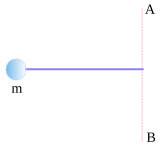Known :

The axis of rotation at AB

Mass ball (m) = 100 gram = 100/1000 = 0.1 kg

The distance between ball and the axis rotation (r) = 30 cm = 0.3 m

Wanted: Moment of inertia of ball (I)

Solution :

I = m r2 = (0.1 kg)(0.3 m)2

I = (0.1 kg)(0.09 m2)

I = 0.009 kg m2

[irp]

2. A 100-gram ball, m1, and a 200-gram ball, m2, connected by a rod with a length of 60 cm. The mass of the rod is ignored. The axis of rotation located at the center of the rod. What is the moment of inertia of the balls about the axis of rotation?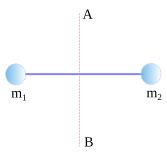Known :

Mass of ball 1 (m1) = 100 gram = 100/1000 = 0.1 kg

The distance of ball 1 and the axis of rotation (r1) = 30 cm = 30/100 = 0.3 m

Mass of ball (m2) = 200 gram = 200/1000 = 0.2 kg

The distance of ball 2 and the axis of rotation (r2) = 30 cm = 30/100 = 0.3 m

Wanted : moment of inertia of the balls

Jawab :

I = m1 r12 + m2 r22

I = (0.1 kg)(0.3 m)2 + (0.2 kg)(0.3 m)2

I = (0.1 kg)(0.09 m2) + (0.2 kg)(0.09 m2)

I = 0.009 kg m2 + 0.018 kg m2

I = 0.027 kg m2

[irp]

3. A 200-gram ball, m1 and a 100-gram ball, m2, connected by a rod with length of 60 cm. Ignore rod’s mass. The axis of rotation located at ball m2. What is the moment of inertia of the balls. Ignore rod’s mass.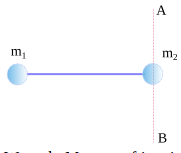Known :

Mass of ball 1 (m1) = 200 gram = 200/1000 = 0.2 kg

The distance between ball 1 and the axis of rotation (r1) = 60 cm = 60/100 = 0.6 m

Mass of ball 2 (m2) = 100 gram = 100/1000 = 0.1 kg

The distance between ball 2 and the axis of rotation (r2) = 0 m

Wanted : Moment of inertia of the balls

Solution :

I = m1 r12 + m2 r22

I = (0.2 kg)(0,6 m)2 + (0.2 kg)(0)2

I = (0.2 kg)(0.36 m2) + 0

I = 0.072 kg m2

[irp]

4. The mass of each ball is 100 gram, connected by cord. The length of cord is 60 cm and the width of cord is 30 cm. What is the moment of inertia of the balls about the axis of rotation. Ignore cord’s mass.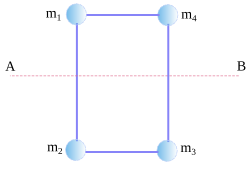Known :

Mass of ball = m1 = m2 = m3 = m4 = 100 gram = 100/1000 = 0.1 kg

The distance between ball and the axis of rotation (r1) = 30 cm = 30/100 = 0.3 m

The distance between ball 2 and the axis of rotation (r2) = 30 cm = 30/100 = 0.3 m

The distance between ball 3 and the axis of rotation (r3) = 30 cm = 30/100 = 0.3 m

The distance between ball 4 and the axis of rotation (r4) = 30 cm = 30/100 = 0.3 m

Known : Moment of inertia

Solution :

I = m1 r12 + m2 r22 + m3 r32 + m4 r42

I = (0.1 kg)(0.3 m)2 + (0.1 kg)(0.3 m)2 + (0.1 kg)(0.3 m)2 + (0.1 kg)(0.3 m)2

I = (0.1 kg)(0.09 m2) + (0.1 kg)(0.09 m2) + (0.1 kg)(0.09 m2) + (0.1 kg)(0.09 m2)

I = 0.036 kg m2

[irp]

The moment of inertia of rigid object

5. What is the moment of inertia of a 2-kg long uniform rod with length of 2 m. The axis of rotation located at the center of the rod.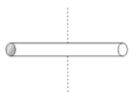Known :

Mass of rod (M) = 2 kg

The length of rod (L) = 2 m

Wanted: Moment of inertia

Solution :

The formula of the moment of inertia when the axis of rotation located at the center of long uniform rod :

I = (1/12) M L2

I = (1/12) (2 kg)(2 m)2

I = (1/12) (2 kg)(4 m2)

I = (1/12)(8 kg m2)

I = 8/12 kg m2

I = 2/3 kg m2

[irp]

6. What is the moment of inertia of a 2-kg long uniform rod with a length of 2 m? The axis of rotation located at one end of the rod.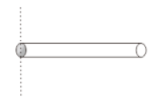Known :

Mass of rod (M) = 2 kg

The length of rigid rod (L) = 2 m

Wanted: Moment of inertia

Solution :

The formula of the moment of inertia when the axis of rotation located at one end of the rod :

I = (1/3) M L2

I = (1/3) (2 kg)(2 m)2

I = (1/3) (2 kg)(4 m2)

I = (1/3)(8 kg m2)

I = 8/3 kg m2

[irp]

7. A 10-kg solid cylinder with a radius of 0.1 m. The axis of rotational located at the center of the solid cylinder, shown in the figure below. What is the moment of inertia of the cylinder?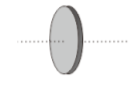Known :

Mass of solid cylinder (M) = 10 kg

Radius of cylinder (L) = 0.1 m

Wanted: The moment of inertia

Wanted: The moment of inertia

Solution :

The formula of moment inertia when the axis of rotation located at the center of cylinder :

I = (1/2) M R2

I = (1/2) (10 kg)(0.1 m)2

I = (1/2) (10 kg)(0.01 m2)

I = (1/2)(0.1 kg m2)

I = 0.05 kg m2

8. A 20-kg uniform sphere with the length of 0.1 m. The axis of rotation located at the center of the sphere shown in the figure below.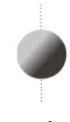Known :

Mass of sphere (M) = 20 kg

The radius of sphere (L) = 0.1 m

Wanted: a moment of inertia

Solution :

The formula of the moment of inertia when the axis of rotation located at the center of the sphere :

I = (2/5) M R2

I = (2/5)(20 kg)(0.1 m)2

I = (2/5)(20 kg)(0.01 m2)

I = (2/5)(0.2 kg m2)

I = 0.4/5 kg m2

I = 0.08 kg m2

[irp]

9. A 2-kg rectangular thin plate with a length of 0.5 m and width of 0.2 m. The axis of rotation located at the center of the rectangular plat shown in the figure below. What is the moment of inertia of the rectangular?

Known :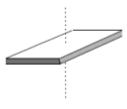Mass of rectangular plat (M) = 2 kg

The length of plat (a) = 0.5 m

The width of plat (b) = 0.2 m

Wanted : Moment of inertia

Solution :

Formula of moment of inertia when the axis of rotation located at the center of plat :

I = (1/12) M (a2 + b2)

I = (1/12)(2)(0.52 + 0.22)

I = (2/12)(0.25 + 0.04)

I = (1/6)(0.29)

I = 0.29/6 kg m2